Courses

# NCERT Solutions Chapter 6 - Lines And Angles (I), Class 9, Maths Class 9 Notes | EduRev

Created by: Indu Gupta

## Class 9 : NCERT Solutions Chapter 6 - Lines And Angles (I), Class 9, Maths Class 9 Notes | EduRev

The document NCERT Solutions Chapter 6 - Lines And Angles (I), Class 9, Maths Class 9 Notes | EduRev is a part of Class 9 category.
All you need of Class 9 at this link: Class 9

Exercise 6.1

1. In Fig. 6.13, lines AB and CD intersect at O. If ∠AOC  ∠BOE = 70° and ∠BOD = 40°, find ∠BOE and reflex ∠COE
.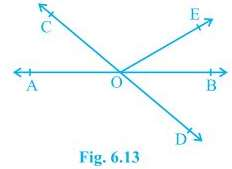∠AOC + ∠BOE = 70° and ∠BOD = 40°
A/q,
∠AOC + ∠BOE +∠COE = 180° (Forms a straight line)
⇒ 70° +∠COE = 180°
⇒ ∠COE = 110°
also,
∠COE +∠BOD + ∠BOE = 180° (Forms a straight line)
⇒ 110° +40° + ∠BOE = 180°
⇒ 150° + ∠BOE = 180°
⇒ ∠BOE = 30°

2. In Fig. 6.14, lines XY and MN intersect at O. If ∠POY = 90° and a : b = 2 : 3, find c.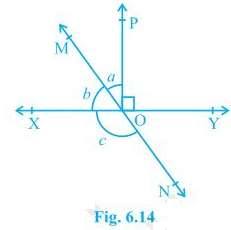Given,
∠POY = 90° and a : b = 2 : 3
A/q,
∠POY + a + b = 180°
⇒ 90° + a + b = 180°
⇒ a + b = 90°
Let a be 2x then will be 3x
2x + 3x = 90°
⇒ 5x = 90°
⇒ x = 18°
∴ a = 2×18° = 36°
and b = 3×18° = 54°
also,
b + c = 180° (Linear Pair)
⇒ 54° + c = 180°
⇒ c = 126°

3. In Fig. 6.15, ∠PQR = ∠PRQ, then prove that ∠PQS = ∠PRT.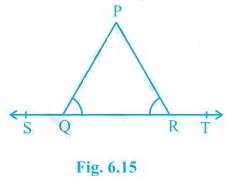Given,
∠PQR = ∠PRQ
To prove,
∠PQS = ∠PRT
A/q,
∠PQR +∠PQS = 180° (Linear Pair)
⇒ ∠PQS = 180° - ∠PQR --- (i)
also,
∠PRQ +∠PRT = 180° (Linear Pair)
⇒ ∠PRT = 180° - ∠PRQ
⇒ ∠PRQ = 180° - ∠PQR --- (ii) (∠PQR = ∠PRQ)
From (i) and (ii)
∠PQS = ∠PRT = 180° - ∠PQR
Therefore,  ∠PQS = ∠PRT

4. In Fig. 6.16, if x y = w z, then prove that AOB is a line.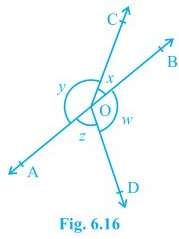Given,
x + y = w + z
To Prove,
AOB is a line or x + y = 180° (linear pair.)
A/q,
x + y + w + z = 360° (Angles around a point.)
⇒ (x + y) +  (w + z) = 360°
⇒ (x + y) +  (x + y) = 360° (Given x + y = w + z)
⇒ 2(x + y) = 360°
⇒ (x + y) = 180°
Hence, x + y makes a linear pair. Therefore, AOB is a straight line.

5. In Fig. 6.17, POQ is a line. Ray OR is perpendicular to line PQ. OS is another ray lying between rays OP and OR. Prove that ∠ROS = 1/2(∠QOS – ∠POS).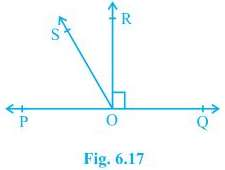Given,
OR is perpendicular to line PQ
To prove,
∠ROS = 1/2(∠QOS – ∠POS)
A/q,
∠POR = ∠ROQ = 90° (Perpendicular)
∠QOS = ∠ROQ + ∠ROS = 90° + ∠ROS --- (i)
∠POS = ∠POR - ∠ROS = 90° - ∠ROS --- (ii)
Subtracting (ii) from (i)
∠QOS - ∠POS = 90° + ∠ROS - (90° - ∠ROS)
⇒ ∠QOS - ∠POS = 90° + ∠ROS - 90° + ∠ROS
⇒ ∠QOS - ∠POS = 2∠ROS
⇒ ∠ROS = 1/2(∠QOS – ∠POS)
Hence, Proved.

6. It is given that ∠XYZ = 64° and XY is produced to point P. Draw a figure from the given information. If ray YQ bisects ∠ZYP, find ∠XYQ and reflex ∠QYP.

Given,
∠XYZ = 64°
YQ bisects ∠ZYP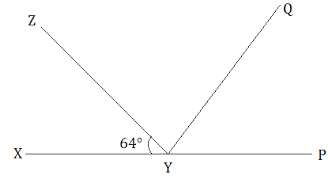∠XYZ +∠ZYP = 180° (Linear Pair)
⇒ 64° +∠ZYP = 180°
⇒ ∠ZYP = 116°
also, ∠ZYP = ∠ZYQ + ∠QYP
∠ZYQ = ∠QYP (YQ bisects ∠ZYP)
⇒ ∠ZYP = 2∠ZYQ
⇒ 2∠ZYQ = 116°
⇒ ∠ZYQ = 58° = ∠QYP
Now,
∠XYQ = ∠XYZ + ∠ZYQ
⇒ ∠XYQ = 64° + 58°
⇒ ∠XYQ = 122°
also,
reflex ∠QYP = 180° + ∠XYQ
∠QYP = 180° + 122°
⇒ ∠QYP = 302°

Exercise 6.2

1. In Fig. 6.28, find the values of x and y and then show that AB || CD.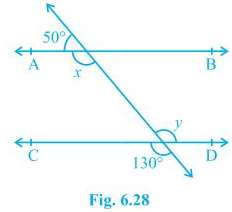x + 50° = 180° (Linear pair)
⇒ x = 130°
also,
y = 130° (Vertically opposite)
Now,
x = y = 130° (Alternate interior angles)
Alternate interior angles are equal.
Therefore, AB || CD.

2. In Fig. 6.29, if AB || CD, CD || EF and y : z = 3 : 7, find x.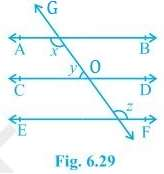Given,
AB || CD and CD || EF
y : z = 3 : 7
Now,
x + y = 180° (Angles on the same side of transversal.)
also,
∠O = z (Corresponding angles)
and, y + ∠O = 180° (Linear pair)
⇒ y + z = 180°
A/q,
y = 3w and z = 7w
3w + 7w = 180°
⇒ 10 w = 180°
⇒ w = 18°
∴ y = 3×18° = 54°
and, z = 7×18° = 126°
Now,
x + y = 180°
⇒ x + 54° = 180°
⇒ x = 126°

3. In Fig. 6.30, if AB || CD, EF ⊥ CD and ∠GED = 126°, find ∠AGE, ∠GEF and ∠FGE.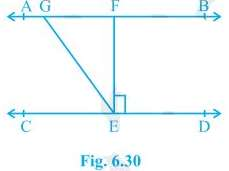Given,
AB || CD
EF ⊥ CD
∠GED = 126°
A/q,
∠FED = 90° (EF ⊥ CD)
Now,
∠AGE = ∠GED (Since, AB || CD and GE is transversal. Alternate interior angles.)
∴ ∠AGE = 126°
Also, ∠GEF = ∠GED - ∠FED
⇒ ∠GEF = 126° - 90°
⇒ ∠GEF = 36°
Now,
∠FGE +∠AGE = 180° (Linear pair)
⇒ ∠FGE = 180° - 126°
⇒ ∠FGE = 54°

4. In Fig. 6.31, if PQ || ST, ∠PQR = 110° and ∠RST = 130°, find ∠QRS.
[Hint : Draw a line parallel to ST through point R.]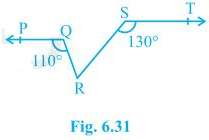Given,

PQ || ST, ∠PQR = 110° and ∠RST = 130°

Construction,

A line XY parallel to PQ and ST is drawn.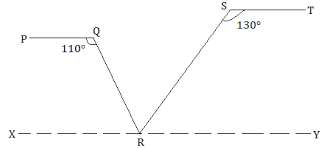∠PQR + ∠QRX = 180° (Angles on the same side of transversal.)
⇒ 110° + ∠QRX = 180°
⇒ ∠QRX = 70°
Also,
∠RST + ∠SRY = 180° (Angles on the same side of transversal.)
⇒ 130° + ∠SRY = 180°
⇒ ∠SRY = 50°
Now,
∠QRX +∠SRY + ∠QRS = 180°
⇒ 70° + 50° + ∠QRS = 180°
⇒ ∠QRS = 60°

5. In Fig. 6.32, if AB || CD, ∠APQ = 50° and ∠PRD = 127°, find x and y.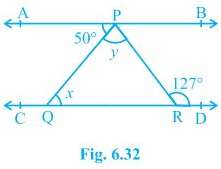AB || CD, ∠APQ = 50° and ∠PRD = 127°
A/q,
x = 50° (Alternate interior angles.)
∠PRD + ∠RPB = 180° (Angles on the same side of transversal.)
⇒ 127° + ∠RPB = 180°
⇒ ∠RPB = 53°
Now,
y + 50° + ∠RPB = 180° (AB is a straight line.)
⇒ y + 50° + 53° = 180°
⇒ y + 103° = 180°
⇒ y = 77°

6. In Fig. 6.33, PQ and RS are two mirrors placed parallel to each other. An incident ray AB strikes the mirror PQ at B, the reflected ray moves along the path BC and strikes the mirror RS at C and again reflects back along CD. Prove that AB || CD.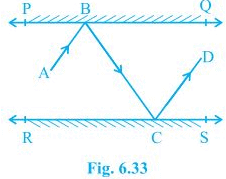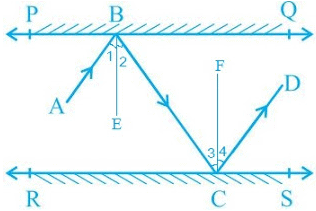Let us draw BE ⟂ PQ and CF ⟂ RS.

As PQ || RS
So, BE || CF

By laws of reflection we know that,

Angle of incidence = Angle of reflection

Thus, ∠1 = ∠2 and ∠3 = ∠4  --- (i)

also, ∠2 = ∠3     (alternate interior angles because BE || CF and a transversal line BC cuts them at B and C)    --- (ii)

From (i) and (ii),

∠1 + ∠2 = ∠3 + ∠4

⇒ ∠ABC = ∠DCB

⇒ AB || CD      (alternate interior angles are equal)

,

,

,

,

,

,

,

,

,

,

,

,

,

,

,

,

,

,

,

,

,

,

,

,

,

,

,

;